Download Presentation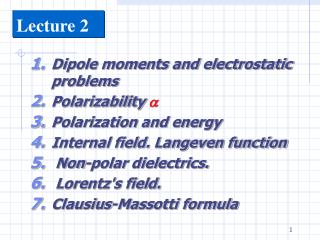Lecture 2

# Lecture 2 - PowerPoint PPT Presentation

Lecture 2. Dipole moments and electrostatic problems Polarizability  Polarization and energy Internal field. Langeven function Non-polar dielectrics. Lorentz's field. Clausius-Massotti formula. Dipole moments and electrostatic problems .I am the owner, or an agent authorized to act on behalf of the owner, of the copyrighted work described.
Download Presentation## Lecture 2

An Image/Link below is provided (as is) to download presentation

Download Policy: Content on the Website is provided to you AS IS for your information and personal use and may not be sold / licensed / shared on other websites without getting consent from its author.While downloading, if for some reason you are not able to download a presentation, the publisher may have deleted the file from their server.

- - - - - - - - - - - - - - - - - - - - - - - - - - E N D - - - - - - - - - - - - - - - - - - - - - - - - - -
Presentation Transcript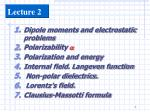Lecture 2
• Dipole moments and electrostatic problems
• Polarizability 
• Polarization and energy
• Internal field. Langeven function
• Non-polar dielectrics.
• Lorentz's field.
• Clausius-Massotti formula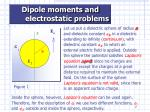Dipole moments and electrostatic problems

Let us put a dielectric sphere of radius aand dielectric constant 2, in a dielectric extending to infinity (continuum), with dielectric constant 1, to which an external electric field is applied. Outside the sphere the potential satisfies Laplace's equation=0,since no charges are present except the charges at a great distance required to maintain the external field. On the surface of the sphere Laplace's equation is not valid, since there is an apparent surface charge.

Figure 1

Inside the sphere, however, Laplace's equation can be used again. Therefore, for the description of , we use two different functions, 1 and 2, outside and inside the sphere, respectively.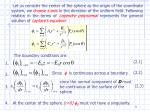(2.1)

Since  is continuous across a boundary

since the normal component ofDmust be continuous at the surface of the sphere

(2.3)

Let us consider the center of the sphere as the origin of the coordinate system, we choose z-axis in the direction of the uniform field. Following relation in the terms of Legendre polynomial represents the general solution of Laplace’s equation:

The boundary conditions are:

1.

(2.2)

2.

3.

4.

At the center of the sphere (r=0) 2 must not have a singularity.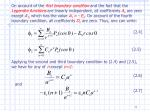(2.4)

(2.5)

(2.6)

(2.7)

On account of the first boundary condition and the fact that the Legendre functions are linearly independent, all coefficients An are zero except A1, which has the value A1 = - Eo. On account of the fourth boundary condition, all coefficients Dnare zero. Thus, one can write:

Applying the second and third boundary condition to (2.4) and (2.5), we have for any of nexcept n=1:

and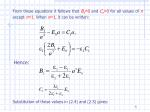From these equations it follows that Bn=0 and Cn=0 for all values of n exceptn=1. Whenn=1, it can be written:

Hence:

Substitution of these values in (2.4) and (2.5) gives: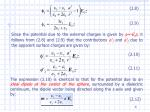(2.8)

(2.9)

(2.10)

(2.11)

(2.12)

Since the potential due to the external charges is given by =-Eoz, it follows from (2.8) and (2.9) that the contributions '1 and '2 due to the apparent surface charges are given by:

The expression (2.10) is identical to that for the potential due to an ideal dipole at the center of the sphere, surrounded by a dielectric continuum, the dipole vector being directed along the z-axis and given by: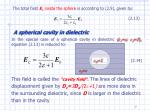(2.13)

2=1

(2.14)

1=

The total field E2inside the sphere is according to (2.9), given by:

A spherical cavity in dielectric

In the special case of a spherical cavity in dielectric (1=; 2=1), equation (2.13) is reduced to:

This field is called the "cavity field".The lines of dielectric displacement given by Dc=3Do/(2+1) are more dens in the surrounding dielectric, since D is larger in the dielectric than in the cavity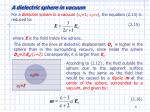(2.15)

2=

1=1

(2.16)

A dielectric sphere in vacuum

For a dielectric sphere in a vacuum (1=1; 2=), the equation (2.13) is reduced to:

where Eis the field inside the sphere.

The density of the lines of dielectric displacement Dsis higher in the sphere than in the surrounding vacuum, since inside the sphere Ds=3Eo/(+2). Consequently, it is larger than Eo.

According to (2.12) , the field outside the sphere due to the apparent surface charges is the same as the field that would be caused by a dipolem at the center of the sphere, surrounded by a vacuum, and given by: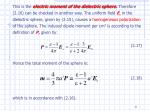(2.17)

(2.18)

This is the electric moment of the dielectric sphere. Therefore (2.16) can be checked in another way. The uniform field Es in the dielectric sphere, given by (2.15), causes a homogeneous polarization of the sphere. The induced dipole moment per cm3 is according to the definition ofP, given by:

Hence the total moment of the sphere is:

which is in accordance with (2.16).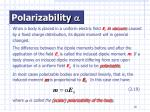(2.19)

Polarizability 

When a body is placed in a uniform electric field Eoin vacuum, caused by a fixed charge distribution, its dipole moment will in general changed.

The difference between the dipole moments before and after the application of the field Eo is called the induced dipole moment m. If a body shows an induced dipole moment differing from zero upon application of a uniform field Eo, it is said to bepolarizable.

In most cases polarizable bodies are polarized linearly, that is, the induced moment m is proportional to Eo. In this case one have:

where is called the (scalar) polarizability of the body.(2.20)

(2.21)

(2.12)

From the dimensions of the dipole moment, [e][l], and the field intensity, [e][l]-2, it follows that the polarizability has the dimension of a volume. Using the above definition of the polarizability, we conclude from equation (2.12) that a dielectric sphere of dielectric constant  and with radius a has a polarizability:

For a conducting sphere in the case from the relation (2.20) one can obtain that a conducting sphere with radius a has a polarizability:(2.22)

There is the fundamental difference between the two types of polarization. In the case of dielectric sphereevery volume element is polarized, whereas in the case of a conducting sphere the induced dipole moment arises from true surface charges.

In some simple cases of spherical molecules, it is possible with  to describe the induced polarization. In general, it is not true and we have to use a polarizability tensor provided the effects remain linear. It leads to:

In such a case the induced dipole moment need to have the same direction as the applied field. In general this direction will depend on the position of the body relative to the polarizing field.(2.23)

(2.24)

(2.25)

Polarization and Energy

Very often it is useful to collect some of the elementary charges into a group forming an atom, a molecule, a unite cell of a crystal, or some larger unit. Let the jth unit of this type contain the selementary charges ej1, ej2,....., ejk,.....,ejs, and let

be an abbreviation for the set of all their displacements rj1,....., rjs.

Then

is the electric moment of this jth group of charges, and

where the sum extends over all the groups.The vector sum of their individual moments m(xj) thus forms the total momentM(X). The main aim of this part is to find the average displacements, and hence the average electric moment under the influence of an external electric field.

In order to obtain a preliminary idea about the average contributions of certain displacements to the electric moment we shall consider two cases, each of which has its characteristic type of displacement:

• The displaced charge is bound elasticity to an equilibrium position; (induced dipole moment)

2) A charge has several equilibrium positions, each of which it occupies with a probability which depends on the strength of an external field.(Permanent dipole moment)

In the first case the displacement of the charge e, carried out by a particle of mass m on a distance r, restoring force proportional to-r acts on the particle in a direction opposite to the displacement (hence the - sign). Thus if the constant external field E is applied:(2.27)

(2.28)

(2.26)

where o/2 denotes the frequency of oscillation, and -mo2ris the restoring force. Equation (2.26) can be written

where

i.e. dr'/dt=0. The charge e, therefore, carries out harmonic oscillations about the position r'which thus represents the time average of its displacement, i.e. if Cand  are constant(2.29)

The average electric moment (induced) is, therefore,

For an example of the second mechanism of polarization let us consider a particle with the chargee that can be in two different equilibrium position Aand B, separated by a distance b. In the absence of an electric field the particle has the same energy in each position.

Thus, it may be assumed to move in a potential field of the type shown in Fig.2.2. In equilibrium with its surroundings it will oscillate with an energy of order kT about either of the equilibrium positions, say about A.(2.30)

Occasionally, however, through a fluctuation, it will be acquire sufficient energy to jump over the potential wall separating it from B. On the time of average, therefore, it will stay in A as long as in B, i.e. the probability of finding it in either A or Bis 0.5.

Fig.2.2

The presence of a fieldEwill affect this in two ways. Firstly, as in case (1), the equilibrium position will be shifted by amount r'which for simplicity will be assumed to be the same in A and B. Secondly, the potential energies VA,VB of the particle in the two equilibrium positions will be altered because its interaction energy with the external field differs by e(bE), i.e.(2.31)

(2.32)

(2.33)

Therefore, in average, the particle should spend more time near B than near A. Actually, since according to statistical mechanics, the probability of finding a particle with energy V is proportional to e-V/kT,

are the probabilities for positions A and B respectively. They have been normalized in such a way as to make

in agreement with the physical condition that the particle must be in one of the two positions. Thus from (2.31) and (2.30)

It follows from the definition of the probabilities pA and pB that if the condition of the system over a long time t1 is considered, the particle will spend a time (use 2.32)(2.34)

(2.35)

(2.36)

in position A, and

in position B. It has thus been displaced by the distance b from A to B during the fraction 1/2(pB-pA) of the time t1. The average momentinduced by the field is thus

Hence, if  is the angle between bandE, the projection of the induced moment into the field direction is, using (2.34) and (2.33) given by

In most cases it is permissible to assume(2.37)

for putting e=4.810-10 e.s.u.,E=300 v/cm.=1 e.s.u., b=10-8 cm. distance between neighboring atoms in a molecule, and T=300oC (room temperature) one finds

Developing (2.35) in terms of ebE/kT, the average induced moment in the field direction is found to be

where er' is a term similar to those considered in case (1) which has been added to account for the elastic displacement. Often two charges +e and -e are strongly bound, forming an electric dipole =ea, wherea is the distance between them. The above case (2) then leads to the same as that of a dipole  having two equilibrium positions with opposite dipole direction, but with equal energy in the absence of a field.(2.38)

(2.39)

(2.40)

In a fieldE the energy of interaction between field and dipole is given by

so that 2Ecos is the energy between the two positions if is the angle andE. This equivalent to equation (2.30) if

Actually putting an immobile charge -ehalfway between A and B turns case (2) into the present case. Clearly the induced moment must be the same for both cases because the charge -e is immobile, and its distance from A and B is 1/2 b, leading to a dipole . Introducing (2.39) into (2.37) yields for the induced moment in the field direction

In contrast to case (1) the electric moment (orientation polarization) now depends on temperature. A matter consisting of a great number of such dipoles will have a temperature-dependent dielectric permittivity in contrast to a substance in which all charges are bound elastically.This means that in thedipolar case the entropy of the substance is decreased by the field.

The difference between the action of the field in the two cases (1) and (2) is essential for the whole theory of dielectric permittivity.

In the case (1), the field exerts a force on elastically bound charge, thus shifting its equilibrium position. In case (2) this force of the field on the charge again leads to contribution of type (1) denoted by er' in equations (2.37) and (2.40). It would be wrong, however, to assume that the field by this force turns a dipole from one equilibrium position into another. This is affected in a more indirect way because the field slightly alters the probabilities of a jump of a dipole from one equilibrium position to another.Internal field; Langeven function

In dielectric two essentially different types of interaction forces should be distinguished. Forces due to chemical bonds, van der Waals attraction, repulsion forces, and others have all such short ranges that usually interaction between nearest neighbors only need be considered. Compared with these forces dipolar interaction forces have a very long range.

As it was shown abovea polarized dielectric can be considered as composed of small regions each having a certain dipole moment, and the total dipole moment of the body is the vector sum of the moments of these regions. It is also known from macroscopic theory that theenergy per unit volume of a macroscopic specimen depends on its shape. This implies that interaction between dipoles must be taken into account even at macroscopic distances.

Due to the long range of the dipolar forces an accurate calculation of the interaction of a particular dipole with all other dipoles of a specimen would be very complicated.A very good approximation can be made by considering that the dipoles beyond a certain distance, say amcan be replaced by a continuous medium, having the macroscopic dielectric properties of the specimen.

Thus the dipole whose interaction with the rest of the specimen we are calculating may be considered as surrounded by a sphere of radius am containing a discrete number of particles, beyond which there is a continuous mediumTo make this a good approximation the dielectric properties of the whole region within the sphereshould be equal to those of a macroscopic specimen, i.e. it should contain a sufficient number of molecules to make fluctuations very small.

Lorentz's methodfor the treatment of dipolar interaction: from a macroscopic specimen select a microscopic spherical region, which is sufficiently large to have the same dielectric properties as a macroscopic specimen. The interaction between the dipoles inside the spherical region will then be calculated in an exact way, but for the calculation of their interaction with the rest of the specimen the letter is considered as a continuous medium.(2.41)

(2.42)

We shall now investigate the dependence of the polarization on the electric fields working on a single molecule. For the induced polarization Pwe write:

where N is the number of particles per cm3,  the scalar polarizability of a particle and Eithe average field strength acting upon that particle. The index k refers to the k-th kind of particle.

The field Eiis called the internal field.It is defined as the total electric field at the position of the particle minus the field due to the particle itself.The calculation of Eiis one of the important problems associated with the theory of dielectrics. This calculation can be executed both in the continuum approach for the environment of the molecule and with the help of statistical mechanics.

Theorientation polarization can be written as:

wherekis the value of the permanent dipole vector averaged over all orientations.(2.43)

The value k can be computed from the energy of the permanent dipole in the electric field. This energy is dependent on the part of the electric field tending to direct the permanent dipoles. This part of the field is called the directing field Ed.

The dependence of k onEd is computed from the energy of dipole in electric field as we did above:

where  is the angle between the directions of Ed and. Since W is the only part of the energy, which depends on the orientation of the dipole, the relative probabilities of the various orientations of the dipole depend on this energy W according to Boltsmann’s distribution law.

Let us consider the average value of <cos>. For a random distribution of the dipoles in matter we have <cos>=0, whereas <cos>=1 if all the dipoles have the same direction as Ed.

From Boltzsmann's law(2.44)

where

and

Fig.2.3

(2.45)

L(a)is called Langeven function

In Fig.2.3 the Langeven function L(a) is plotted against a.L(a)has a limiting value 1, which was to be expected since this is the maximum of cos. For small values of a, <cos> is linear in Ed:(2.46)

(2.47)

The approximation of equation (2.45) may be used as long as

At room temperature (T=300o K) this gives for a dipole of 4D:

= 3 105 v/cm

For a value of smaller than the large value of 4D, the value calculated for Ed is even larger. In usual dielectric measurements, Ed is much smaller than 105 v/cm and the use of (2.45) is allowed.

From equation (2.45) it follows that:

Substituting this into (2.42) we get:

From the first lecture we had(2.48)

(2.49)

We now substitute (2.41) and (2.47) into (2.48) and find:

This is the fundamental equation is the starting point for expressing Eiand Ed as functions of the Maxwell field E and the dielectric constant .(2.50)

(2.14)

For a non-polar system the fundamental equation for the dielectric permittivity (2.49) is simplified to:

In this case, only the relation between the internal field and the Maxwell field has to be determined.

Let us use the Lorentz approach in this case. He calculated the internal field in homogeneously polarized matter as the field in a virtual spherical cavity.

The field in such a cavity differs from the field in a real cavity, given by (2.14), because in the latter case the polarization adjusts itself to the presence of the cavity.

Therefore the polarization in the environment of a real cavity is not homogeneous, whereas the polarization in the environment of a virtual cavity remains homogeneous.Fig.2.4

(2.51)

The field in a virtual spherical cavity, which we call the Lorentz fieldEL, is the sum of:

• the Maxwell field E caused by the external charges and by the apparent charges on the outer surface of the dielectric, and
• the field Esphinduced by the apparent charges on the boundary of the cavity (see fig.2.4).

The field Esph is calculated by subdividing the boundary in infinitesimally small rings perpendicular to the field direction. The apparent surface charge density on the rings is -Pcos, their surface is 2r2sind so that the total charge on each ring amounts to:

According to Coulomb's law, a charge element de tothe field component in the direction of the external field , given by:(2.52)

(2.53)

(2.54)

(2.55)

Combining (2.51) and (2.52), we find for the component of Esphin the direction of the external field :

For the reasons of symmetry, the other components of Esph are zero, and we have with EL=E+Esph:

This is Lorentz's equation for the internal field.

Substituting (2.54) into (2.50), we find:

This relation is generally called the Clausius-Massotti equation.(2.56)

(2.57)

(2.58)

(2.59)

For a pure compound it is reduced to:

From the Clausius-Mossotti equation for a pure compound, it follows that it is useful to define a molar polarization [P]:

where dis the density and M the molecular weight. When the Clausius-Mossotti equation is valid [P] is a constant for a given substance:

The Clausius-Mossotti equation can also be used for polar systems in high-frequency alternating fields:(2.60)

in which is the dielectric constant at a frequency at which the permanent dipoles (i.e. the orientation polarization) can no longer follow the changes of the field but where the atomic and electronic polarization are still the same as in static fields.

Often this equation is used for still higher frequencies, where the atomic polarization too cannot follow the changes of the field. If according to Maxwell relation for the dielectric constant and the refractive index =n2, it is possible to write: